# Brendan Kelly Publishing

Brendan Kelly Publishing works with prominent authors who simplify complex ideas in a clear and entertaining style.  BKP currently publishes a comprehensive suite of products that enable the use of TI Technology in the classroom.

To receive 12 free TI-Nspire files, visit the Brendan Kelly Publishing website at www.brendankellypublishing.com## Algebra I with TI-Nspire™

With ready-made lessons, complete keying sequences, and detailed exercise solutions, Brendan Kelly's latest books help your students understand the fundamental concepts of Algebra I by exploiting the full pedagogical power of TI-Nspire™ technology.

 Semester 1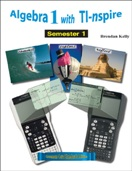Topics include: transitioning from arithmetic to algebra linear equations and inequalities functions and relations linear functions linear systems

 Semester 2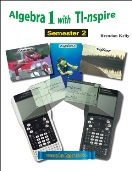Topics include: quadratic functions and equations polynomials data analysis and probability exponential and radical functions rational functions and equations

## Algebra 2 with TI-Nspire™

Designed to help educators implement TI-Nspire technology in the teaching of all the important Algebra 2 concepts, these books focus on the fundamental skills of algebra while allowing students to solve real-world problems using graphs, spreadsheets and the appropriate TI-Nspire commands.

 Semester 1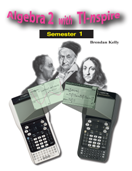Topics include: numbers and number systems sequence, series, and functions matrices linear systems quadratic functions and equations polynomial and polynomial equations

 Semester 2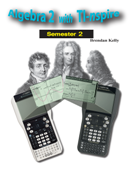Topics include: rational and radical functions and equations exponential and logarithmic functions and equations trigonometric functions and equations trigonometric identities analytic geometry–conics and polar coordinates permutations, combinations and probability

## TI-84 Plus Trilogy

These books allow teachers and students to easily integrate the TI-84 into teaching and learning mathematics. Each exploration in the book begins with a little piece of mathematical history then, through step-by-step explanations and lists of calculator key sequences, with engaging presentations of various topics.

 Functions with TI-84 Plus Calculator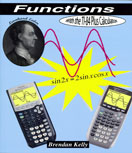Topics include: properties of polynomial functions rational functions trigonometric functions exponential functions logarithmic functions polynomial equations extrema

 Statistics with TI-84 Plus Calculator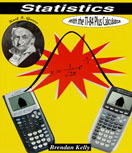Topics include: data graphs scatterplots correlation probability regression analysis binomial distribution normal distribution tests of hypotheses

 Advanced Algebra with TI-84 Plus Calculator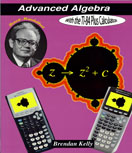Topics include: sequences and series math of investment number theory solving equations probability matrices linear programming fractals and chaos

## TI-89 Trilogy

These books contain carefully designed instructional activities that showcase TI-89 into teaching and learning of important math concepts.  Each text includes ready-made lessons and explorations, complete keying sequences and exercises with detailed solutions.

 Advanced Algebra with the TI-89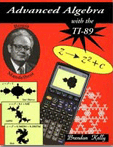Topics include: Fundamental Theorem of Algebra sequences and series polynomial functions polynomial equations rational functions trigonometric functions trigonometric identities exponential functions logarithmic functions

 Statistics with the TI-89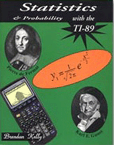Topics include: box plots and data graphs histograms measures of central tendency measures of dispersion scatterplots correlation probability regression analysis lines of best fit

 Calculus with the TI-89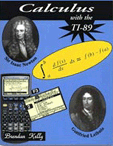Topics include: sequences and series limits derivatives of rational and transcendental functions related rates & motion equations of tangents asymptotic behavior arc lengths of curves differential equations